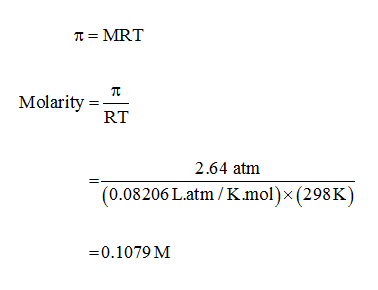# A solutions that is 0.100M in hydrofluoric acid (HF) has an osmotic pressure of 2.64 atm at 13) 25°C. Calculate the percent ionization of HF in this solution

Question
46 views

A solutions that is 0.100M in hydrofluoric acid (HF) has an osmotic pressure of 2.64 atm at 13) 25°C. Calculate the percent ionization of HF in this solution

check_circle

Step 1

Molarity of the solution can be calculated as follows,...

### Want to see the full answer?

See Solution

#### Want to see this answer and more?

Solutions are written by subject experts who are available 24/7. Questions are typically answered within 1 hour.*

See Solution
*Response times may vary by subject and question.
Tagged in

### Chemistry